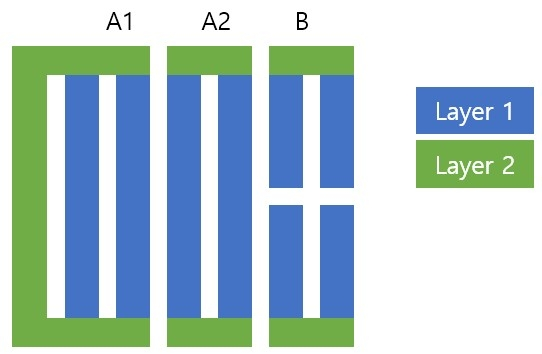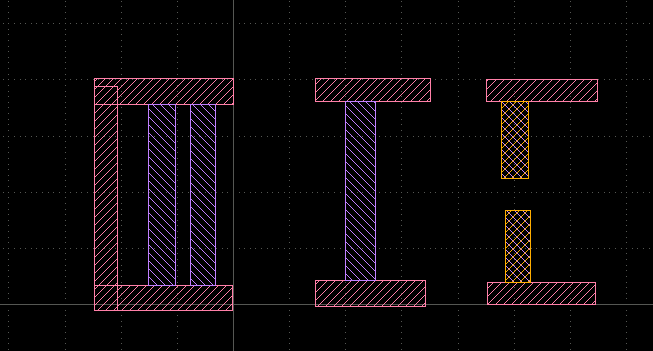# Classify interaction of polygons

edited December 2021I need to classify polygons at Layer 1 according to the number of sides interacting with Layer 2 polygons. (A vs. B.)

`B = layer1.interacting(layer2, 1, 1)`

This code only works for case A2. How can I differentiated A1 vs. B? (I also tried with edges with no success.)

• Yes, case A1 is a interaction with one polygon. So initially it cannot be distinguished from case B.

But you can compute the touching edges (provided you're only interested in touching interactions) and check how many edges are interacting with one polygon of layer 1:

``````l1 = input(1, 0)
l2 = input(2, 0)

touching = l1.edges & l2.edges

l2.interacting(touching, 1..1).output(100, 0)
``````Matthias